# c#写的遗传算法的交叉算子，选择算子和变异算子

遗传算法是一类借鉴生物界的进化规律（适者生存，优胜劣汰遗传机制）演化而来的随机化搜索方法。其主要特点是直接对结构对象进行操作，不存在求导和函数连续性的限定；具有内在的隐并行性和更好的全局寻优能力；采用概率化的寻优方法，能自动获取和指导优化的搜索空间，自适应地调整搜索方向，不需要确定的规则。

#### 1.交叉算子

编码是应用遗传算法时要解决的首要问题，也是设计遗传算法时的一个关键步骤。编码方法除了决定了个体的染色体排列形式之外，还解决了个体从搜索空间的基因型变换到解空间的表现型时的解码方法，编码方法也影响到交叉算子、变异算子等遗传算子的运算方法。二进制编码方法是遗传算法中最常见的一种编码方法，它使用的编码符号集是由二进制符号0和1所组成的二值符号集 {0,1} ，它所构成的个体基因型是一个二进制编码符号串。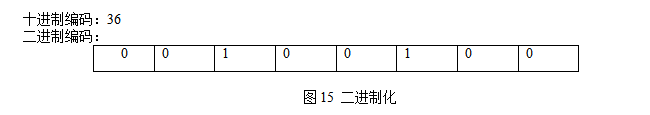遗传算法中的所谓交叉运算，是指对两个相互配对的染色体按某种方式相互交换基因，从而形成新的个体。交叉运算是遗传算法的重要特征，它在遗传算法中起着关键作用，是产生新个体的主要方法。

最常用的交叉算子是单点交叉算法，又称简单交叉，它是指在个体编码串中随机设置一个交叉点，然后在该点相互交换两个配对个体的部分染色体。如图所示：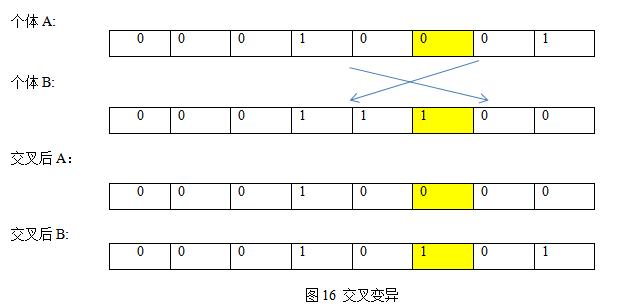int binary(int a, int b)//a,b为要交叉变异的两个数
{
Random rad = new Random();
int radval = rad.Next(0, 5);
int numn = 0, i;
int[] table1 = new int;//定义记录二进制数表
int[] table2 = new int;
for ( i = 7; i >= 0; i--)//将数值模拟为0、1表示的二进制
{
table1[i] = (a>> i) & 0x01;
table2[i] = (b>> i) & 0x01;
}
for (i = 0; i <= radval; i++)//随机交叉进行交叉互换
{
table1[i] = table2[i];
}
for (i = 0; i < 8; i++)//再转换成十进制形式
{
numn += table1[i] * (int)Math.Pow(2, i);
}
return numn;
}


#### 2.选择算子

在生物的遗传和自然进化中，对生存环境适应程度较高的物种将有更多机会遗传到下一代；而对生存环境适应度程度较低的物种遗传到下一代的机会就相对较少。模仿这个过程，遗传算法使用选择算子来对群体中的个体进行优劣淘汰操作：适应度较低的个体被遗传到下一代群体中的概率较小；适应度高的个体遗传到下一代的概率较大。遗传算法的选择操作就是用来确定如何从父代群体中按某种方法选取哪些个体遗传到下一代群体中的一种遗传算法。

最常用的选择算子是基本遗传算法的比例选择算子。比例选择算子是一种回放式随机采样的方法。其基本思想是：各个个体被选中的概率与其适应度大小成正比。设群体大小为M。个体i的适应度为 ，则个体i被选中的概率为: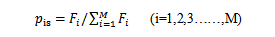由此可见，适应度越高的个体被选中的概率越大。我们用数组的方式模拟这种概率的选择。将适应度根据从小到大的方式存入数组中，排名越靠后，适应度越大，应该使它们被选中的概率更大。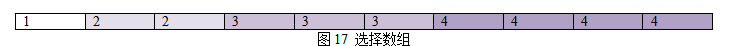数组部分如图所示，数组中的数字代表在特定数值（本例为道路流畅度）由小到大的排名即本例的适应度值，流畅度为1的占1格，2的占2格，以此类推，不同排名值占据其本身数值个格子，排名越高，说明其适应度值越大，占据的格子也越多，被选中的概率也就越大。

int choose()
{
int i, tem = 1, num = 0;
int total = 0;
Random rad = new Random();
for (i = 1; i <= yunxingcanshu.gen; i++)//计算个体的总和
{
total += i;
}
int[] probability = new int[total];//创建选择数组
for (i = 0; i < total; i++)//为数组赋值
{
if (num < tem)
{
probability[i] = tem;
num++;
}
else
{
tem++;
num = 1;
probability[i] = tem;
}
}
int radval = rad.Next(1, total);
return probability[radval] - 1;//返回被选中的排名
}//选择函数


#### 3.变异算子

变异运算是对某一个基因座上的基因值按一较小概率进行改变,它也是产生新个体的一种操作方法.本例中,我们采用基本位变异的方法来进行变异运算,其具体操作过程是:首先确定出各个个体的基因变异位置，然后依照某一概率将变异点的原有基因取反。

例如，若第3号个体的第2个基因座需要进行变异运算，则可产生一个新个体。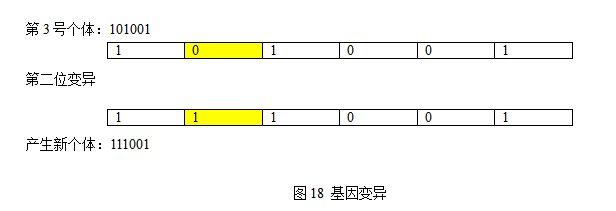int variation(int a)//变异函数
{
Random rad = new Random();
int radval1 = rad.Next(0, 10), radval2 = rad.Next(3, 8);
int num = 0;
int[] table = new int;
int i;
for (i = 7; i >= 0; i--)//转化为二进制形式
{
table[i] = (a>> i) & 0x01;
}
if (radval1 == 0)//以十分之一概率变异
{
table[radval2] = 1 - table[radval2];//交换随机位置二进制值
}
for (i = 0; i < 8; i++)//翻译成十进制
{
num += table[i] * (int)Math.Pow(2, i);
}
return num;//返回经过变异后的数值
}